Next: 4.4.2 Chemical-Mechanical Planarization Up: 4.4 Boolean Operations Previous: 4.4 Boolean Operations

## 4.4.1 Implementation

If the H-RLE data structure is used, Boolean operations can be implemented in a very efficient manner. The minimum or maximum of two LS functions is obtained by moving two basic iterators over the corresponding H-RLE data structures. The common range of both iterators is defined as the maximum of both minimum index vectors and by the minimum of both maximum index vectors. To advance the pair of iterators to the next position, all iterators are moved forward, whose maximum index vector is equal to the maximum index vector of the common range. This is in analogy to the realization of neighbor access using a stencil of iterators, as described in Section 4.2.2.

At every step, the minimum or the maximum LS value of both iterators is calculated. Recalling that the LS value of undefined runs is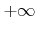or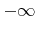(see Section 4.2.1), the result can also take these values. In this case an undefined run is inserted into the new H-RLE data structure using the minimum index vector of the common range. Otherwise, if the result is notor, at least one of the two iterators must be at the position of a defined grid point. Hence, the common range is exactly this single grid point, for which the common minimum and maximum index vector are identical. This index vector is used to add a defined grid point to the H-RLE data structure.

The resulting new H-RLE data structure does not necessarily fulfill (3.30). Hence, grid points belonging to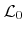do not necessarily have an opposite signed neighbor leading to inefficient sets of active grid points. To avoid dense sets of such nonrelevant grid points they must be removed using a pruning procedure as described in Section 4.3.2.

Overall, the calculation of the minimum or the maximum of two LS functions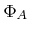and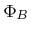can be carried out in linear time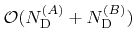, if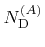and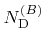denote the corresponding numbers of defined grid points. The determination of the complement requires only the inversion of the sign. This can be performed without rebuilding the H-RLE data structure, by simply changing the sign of all values in the LS array and interchanging the run codes of all positive and negative undefined runs. The application of Boolean operations on H-RLE data structures is demonstrated in Figure 4.4. It shows the relative complement of a sphere in the union of a cuboid and a cone.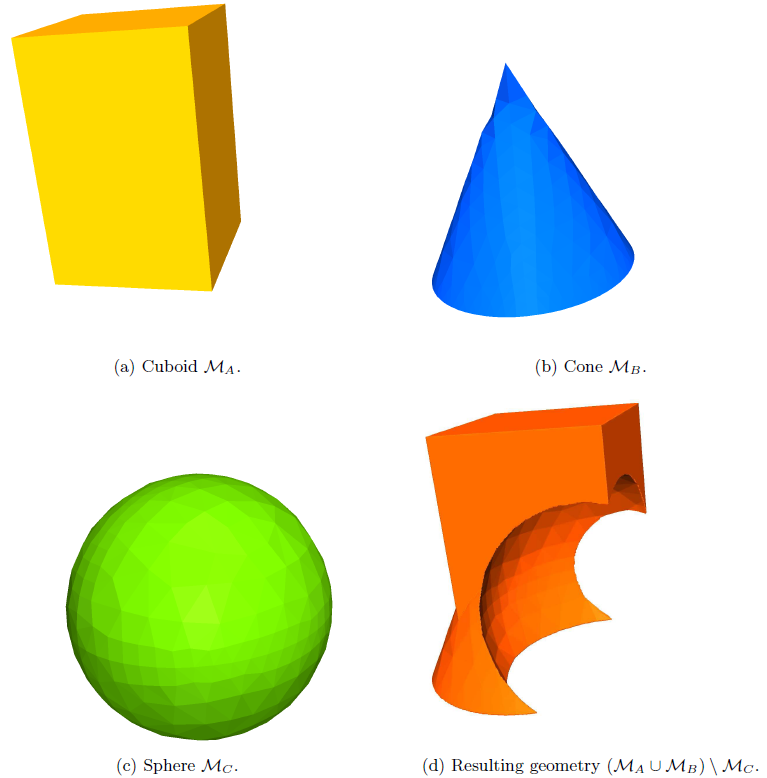Next: 4.4.2 Chemical-Mechanical Planarization Up: 4.4 Boolean Operations Previous: 4.4 Boolean Operations

Otmar Ertl: Numerical Methods for Topography Simulation